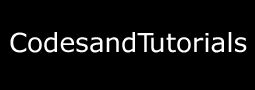Next generation website theme is here (black, neon and white color) - Reduces eye strain and headache problems.

## Digital Codes

Computers and digital circuits processes information in the binary format. Each character is assigned 7 or 8 bit binary code to indicate its character which may be numeric, alphabet or special symbol. Example - Binary number 1000001 represents 65(decimal) in straight binary code, alphabet A in ASCII code and 41(decimal) in BCD code.

### Types of codes

BCD (Binary-Coded Decimal) code :

• Four-bit code that represents one of the ten decimal digits from 0 to 9.
• Example - (37)10 is represented as 0011 0111 using BCD code, rather than (100101)2 in straight binary code.
• Thus BCD code requires more bits than straight binary code.
• Still it is suitable for input and output operations in digital systems.

Note: 1010, 1011, 1100, 1101, 1110, and 1111 are INVALID CODE in BCD code.

ASCII (American Standard Code Information Interchange) code :

• It is 7-bit or 8-bit alphanumeric code.
• 7-bit code is standard ASCII supports 127 characters.
• Standard ASCII series starts from 00h to 7Fh, where 00h-1Fh are used as control characters and 20h-7Fh as graphics symbols.
• 8-bit code is extended ASCII supports 256 symbols where special graphics and math's symbols are added.
• Extended ASCII series starts from 80h to FFh.

EBCDIC (Extended Binary Coded Decimal Interchange Code) code

• 8-bit alphanumeric code developed by IBM, supports 256 symbols.
• It was mainly used in IBM mainframe computers.

Gray code

• Differs from leading and following number by a single bit.
• Gray code for 2 is 0011 and for 3 is 0010.
• No weights are assigned to the bit positions.
• Extensively used in shaft encoders.

Excess-3 code

• 4-bit code is obtained by adding binary 0011 to the natural BCD code of the digit.
• Example - decimal 2 is coded as 0010 + 0011 = 0101 as Excess-3 code.
• It not weighted code.
• Its self-complimenting code, means 1's complement of the coded number yields 9's complement of the number itself.
• Used in digital system for performing substraction operations.

## Tip Box

Error dectecting and correcting schemes

• Repetition codes
• Parity bits
• Checksums
• Cyclic redundancy checks (CRCs)
• Cryptographic hash functions
• Error-correcting codes
• Error correction
• Automatic repeat request
• Error-correcting code
• Hybrid schemes